§9  空间曲线

[曲线的方程与正向]

 曲 线 方 程 的 形 式 曲 线 的 正 向 交面式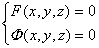参数式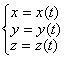或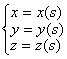（t为任意参数，s为曲线的弧长） 矢量式r = r ( t )或r = r ( s ) （r (t) = x (t) i + y (t) j + z (t) k, t, s同上） t（或s）增加时，曲线上一点运动的方向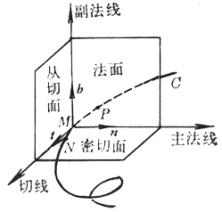图  7.18

[活动标架的三个单位矢量]  t为单位切线矢量，方向与曲线的正向一致；n为单位主法线矢量，它指向曲线的凹方；b为单位副法线矢量，b=t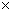n.t,n,b构成右手系（图7.18.这三个矢量称为曲线在点M的活动标架（或叫动标三面形、伴随三面形，也叫活动标形）.

[活动标架所在直线和平面的方程]  M(x0,y0,z0)（图7.18.

切线      过曲线上两点N,M的直线NM，当N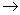M时的极限位置.其方程为

参数式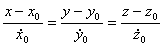（以t为参数）

矢量式 r=r0+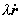（以t为参数）

交面式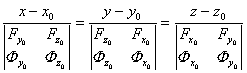法面      与切线垂直的平面（通过M的法面上一切直线都称为曲线在M的法线）.其方程为

矢量式             (r-r0)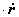=0（以t为参数）

交面式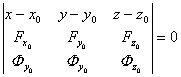密切面  通过曲线上三点M,P,N作一平面，当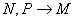时，平面的极限位置（切线在密切面上）.其方程为

参数式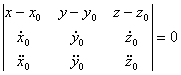（以t为参数）

矢量式 ((r-r0)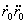)=0（以t为参数）

主法线  法面与密切面的交线.其方程为

l=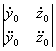,   m=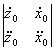, n=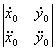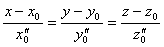（以s为参数）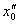表示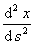在点M的值，等等.

r=r0+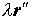（以s为参数）

副法线  垂直于密切面的直线.其方程为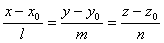（以t为参数）

矢量式

r0=r0+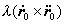（以t为参数）

从切面  通过切线与副法线的平面.其方程为

参数式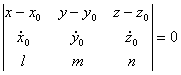（以t为参数）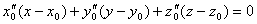（以s为参数）

矢量式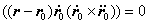（以t为参数）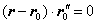（以s为参数）

[曲率与挠率的定义与公式]

 公 式 与 意 义 图    形 曲率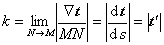曲率半径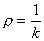k表示包含点M的部分曲线偏离直线的程度，也是切线方向对于弧长的转动率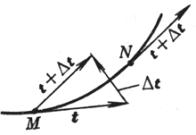挠率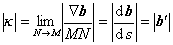挠率半径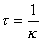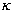表示包含点M的部分曲线偏离平面曲线的程度.=0的曲线是平面曲线.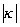是曲线在点M挠率的绝对值，它等于副法线方向对于弧长的转动率.   挠率的符号：当点M沿曲线的正向移动时，矢量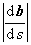与n反向，则取正号，反之取负号（图(b)）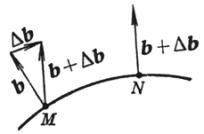(a)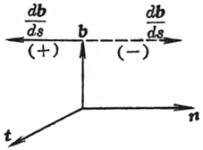(b)

表中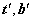分别表示t,bs的导数.

[曲率与挠率的计算公式]

曲率

参数式

k=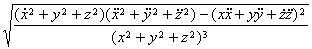（以t为参数）

k=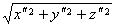（以s为参数）

矢量式

k=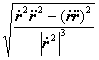（以t为参数）

k=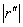（以s为参数）

挠率的绝对值

参数式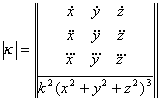（以t为参数）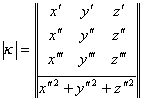（以s为参数）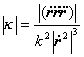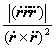（以t为参数）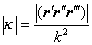（以s为参数）

[雪列-弗莱纳公式（或基本公式）]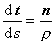,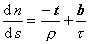,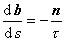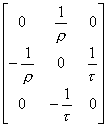这组公式与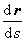=t合并起来描述了点M在曲线上移动时活动标架的运动规律.

把活动标架看作一个刚体，就是当M沿曲线移动时，M的活动标架好象刚体那样绕M转动.这时把s看作时间，则根据运动学的原理可以得出活动标架的瞬时转动速度的表达式为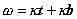曲线的曲率等于活动标架绕着副法线的转动支量，挠率等于绕着切线的转动支量.

最后，由可以验证，空间曲线的雪列-弗莱纳公式就是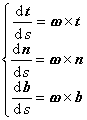这就是雪列-弗莱纳公式的运动学意义.

[基本定理与自然方程]    在一闭区间a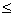sb上给定任意两个连续函数k(s)(s)，其中k(s)>0，则除了空间的位置差别外，唯一地存在一条空间曲线，它以s为弧长，可k(s)为曲率，(s)为挠率.

k=k(s),=(s)